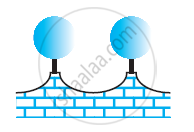Share
Notifications

View all notifications
Books Shortlist
Your shortlist is empty

# The Front Compound Wall of a House is Decorated by Wooden Spheres of Diameter 21 Cm, Find the Cost of Paint Required If Silver Paint Costs 25 Paise per Cm^2 and Black Paint Costs 5 Paise per Cm^2. - CBSE Class 9 - Mathematics

Login
Create free account

Forgot password?
ConceptVolume of a Sphere

#### Question

The front compound wall of a house is decorated by wooden spheres of diameter 21 cm, placed on small supports as shown in the given figure. Eight such spheres are used for this purpose, and are to be painted silver. Each support is a cylinder of radius 1.5 cm and height 7 cm and is to be painted black. Find the cost of paint required if silver paint costs 25 paise per cm2 and black paint costs 5 paise per cm2.#### Solution

Radius (r) of wooden sphere = (21/2)cm = 10.5cm

Surface area of wooden sphere = 4πr2

=[4xx22/7xx(10.5)^2]cm^2 = 1386cm^2

Radius (r1) of the circular end of cylindrical support = 1.5 cm

Height (h) of cylindrical support = 7 cm

CSA of cylindrical support = 2πrh

=[2xx22/7xx(1.5)xx7]cm^2 = 66cm^2

Area of the circular end of cylindrical support = πr2

=[22/7xx(1.5)^2]cm^2=7.07cm^2

Area to be painted silver = [8 × (1386 − 7.07)] cm2

= (8 × 1378.93) cm2 = 11031.44 cm2

Cost for painting with silver colour = Rs (11031.44 × 0.25) = Rs 2757.86

Area to be painted black = (8 × 66) cm= 528 cm2

Cost for painting with black colour = Rs (528 × 0.05) = Rs 26.40

Total cost in painting = Rs (2757.86 + 26.40)

= Rs 2784.26

Therefore, it will cost Rs 2784.26 in painting in such a way.

Is there an error in this question or solution?

#### APPEARS IN

RD Sharma Solution for Mathematics for Class 9 by R D Sharma (2018-19 Session) (2018 to Current)
Chapter 21: Surface Areas and Volume of a Sphere
Ex.21.10 | Q: 13 | Page no. 9
NCERT Solution for Mathematics Class 9 (2018 to Current)
Chapter 13: Surface Area and Volumes
Ex.13.90 | Q: 2 | Page no. 237
Solution The Front Compound Wall of a House is Decorated by Wooden Spheres of Diameter 21 Cm, Find the Cost of Paint Required If Silver Paint Costs 25 Paise per Cm^2 and Black Paint Costs 5 Paise per Cm^2. Concept: Volume of a Sphere.
S